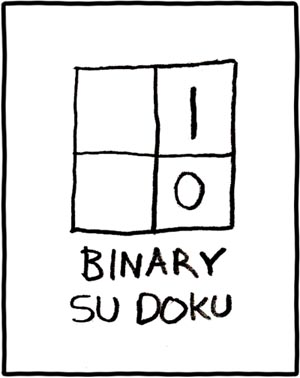# Two Guys Arguing

## A Solution from Purgatory (for the Matrix from Hell)

Posted in challenge, math, questions by benjaminplee on 01.02.11

###This post attempts to recount some of my thought process which eventually (more than a year later) lead to the Matrix from Hell puzzle I posted about a few days ago.  If you don’t want to know my answer, don’t read the rest of this post.  My final solution is at the bottom.

#### You have been warned.

~~~~~~~~~~~~~~~~~~~

~~~~~~~~~~~~~~~~~~~~~~~~~~~~~~~~~~~~~~

~~~~~~~~~~~~~~~~~~~

Below is a list of my general thought process and the main ideas/revelations/halucinations that I know helped me to my solution.  An annotated matrix is at the bottom with some of the ideas highlighted in different colors.

• Should indices start at 0 or 1?
• Values along either axis count up by 1 in both directions when the other =0
• Values are duplicated over the f(x)=y axis –> Order of indices given to function is inmaterial since they can be swapped
[The RED 5 can be seen duplicated over the diagonal]
• Calculation of max/min index is O(1) so stop worry about it and “forget” the bottom half of the matrix (assume X>= Y)
• Values generally increase as they go right/down but not always
• f(x,x)=0, f(x,0)=x
• Values are symmetrical perpendicular to f(x)=y IFF the bounding square in question is a power of 2
• “Quad” patterns can be seen repeating for every power of 2.  In each “Quad” the top left quadrant is the same as the bottom right and the top right is the same as the bottom left.
[Each “Quad” of the example matrix is outlined in BLUE with the quadrants in LIGHT BLUE. PINK shows the repetition]
• power of 2 repeating nature of the values leads to thinking of binary trees
• binary trees leads to thinking about a possible solution which could run in O(log2 n) time:
• In a nut shell, for a given (X,Y), algorithm would find smallest boudning power of 2 including X and would walk back “Quad”s by each successivly smaller power of 2 “Quad” until the last one is met.  For each “Quad” determine which quadrant the value lives in and remap to root values.
• Debate if this would really work for large numbers and if it could be reduced to constant time function by math that I have forgotton from college/HS
• Dismiss b/c I can’t remember a way and base idea is > O(1)
• Binary trace back algorithm idea makes me realize that it can be also looked at as the “binary” representation of a number (e.g. 01011011010) where each 1 represents a “Quad” where the value was in the upper right or bottom left and vica-verca.
[Following the matrix value at (5,6) can be seen ORANGE.  The value 3 is in the bottom right  of the 1st “Quad”, then BL, & TR. {BR BL TR} => {101} => {3}]
• Remembered that f(x,y)=0 IFF x==y …. which means that difference between X and Y is important to value of f()
• Eureka!
• F(X, Y) = X xor Y
• solution typed above in white on white to protect the innocent. highlight to view.

### That is it.  I never said it was pretty or made any sense.

Tagged with: , , , ,

## Matrix Puzzle From Hell or: How I Learned to Stop Worrying and Love the Patterns

Posted in challenge, math by benjaminplee on 12.30.10
#####The Stage

About a year ago, Mr. King (@Adkron) posed a math puzzle on our team’s whiteboard first thing one morning.  The problem seemed at the same time very complex and strangely simple.  Amos said that he had found it surfing the web the night before and that it was from some company’s interview or resume-submitting questions.

After several passionate whiteboarding sessions including members of our team, and any poor souls who walked by (I believe Mr. Young falls into this group), no one had a solution.  Eventually the whiteboard was clearred for more “important” work but the puzzle lingered with me.  On several occasions over the past year I have redrawn the matrix on a pad of paper and spent and hour or two trying to decipher the patterns and make sense of the answer tickling at edge of my brain.

This past week, while drifting off to sleep, the puzzle popped back into my head.  After a few moments a number of ideas I had had months before fell together in just the right way for me to FINALLY figure out a solution.  Eureka! (I mentally shouted) and got out of bed to find a piece of paper to work through some more examples and prove myself right.

## The Puzzle

Given a 2D matrix such that each cell’s value is defined as follows:

>> The smallest non-negative integer not already present to the “left” or “above” <<

Determine an constant time function ( O(1) ) which given X and Y indices will return the value of the matrix cell at (X,Y).

For the visual people in the crowd, the top left corner or said matrix looks something like this:

The picture only shows the top 8×8 of the defined matrix as an example.  We can see that whatever f(x,y) you come up with needs to equal the following example values

f(0,0) = 0
f(1,0) = 1
f(2,0) = 2
f(3,0) = 3
f(3,1) = 2
f(3,2) = 1
……

The real key here is that the solution needs to function in constant time: O(1).  This means that computing the matrix value at (6,3) should be the same cost computationally as computing the matrix value at (10000000,4500005).

Anyone can write a program to compute the whole matrix out to your point (in theory) but can you come up with a better solution? In less than a year?

Tagged with: ,